# Geodesic coordinates

at a pointof a space with an affine connection with connection coefficientsAny system of coordinates in which all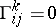at. If the equalityis satisfied at all points of a given curve, one speaks of geodesic coordinates along the curve (Fermi coordinates). In a Riemannian space with metric tensor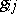, geodesic coordinatesare often defined by the conditions, which in this case are equivalent to the condition. For a symmetric connection, in particular, a Riemannian connection, geodesic coordinates exist at any point and along any regular arc without self-intersections. For a surfacein Euclidean space, geodesic coordinates are given by rectangular Cartesian coordinates of the tangent plane ofat; if the projection is effected onto the developable surface, enveloped by the planes tangent toalong a curve, then the intrinsic Cartesian coordinates onwill be Fermi coordinates on.

In geodesic coordinates the components at a pointof the covariant derivatives of a tensor field atare equal to the ordinary derivatives of the tensor components. This may be taken as the definition of a covariant derivative, following E. Cartan's idea on the transfer of geometric objects or the operations of Euclidean geometry into more general spaces using special coordinate systems in which the effects of their non-Euclidean nature are eliminated to the greatest extent. This idea also forms the base of the use of geodesic coordinates in general relativity theory, where they are connected with locally inertial reference systems in space-time; the study of such systems plays an important role in the physical interpretation of the theory.

Geometric conditionsmean that there is a correspondence between the straight lines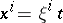(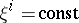,is a parameter) in the domain where the coordinates are defined and curvesin the space under consideration with initial at a point,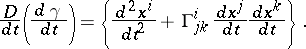If the geodesic coordinates are such that to the straight lines issuing from a pointin all directions there correspond geodesics on which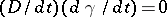, then they are called Riemannian coordinates.